# Power Amplifier Circuit with IC ANxxxx Collection

Power amplifier using IC ANxxxx :
AN7154
This amplifier use IC AN7154 , this is  minimum voltage for circuit is 9 volt and maximum voltage 18 volt with dual suplly voltage. Maximum power output 20 watt with minimum impedance 4 Ohm. This circuit equipped treebel booster , to maximum performance for treebel sound out.
See amplifier schematic below :Click to view enlarge
Voltage : 12 Volt
Output : 20 watt

AN7145L
On this schematic is Stereo Power Amplifier circuit  use the ic AN7145L , but can use the following IC :

 AN7145M AN7145H AN7146M AN7146H

You can use of all ic above. anthis circuit need suply voltage with dual voltage . Minimum voltage 6 Volt and maximum voltage 20 volt . Voltage just (-) and (+), but (-) can also ground. Maximum power output 2 X 20 watt with minimum impedance 4 ohm.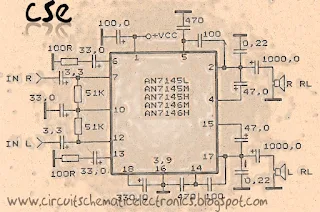Click image to view enlarge.

You can operate this circuit with  plugging input to , PC or other media can give signal audio.

AN7143
On this Integrated  circuit power amplifier use IC AN7143 but you can use this following IC :

 AN7147 HA1377 AN7149N HA1377A AN7168 HA1392 AN7169 HA1398 AN7176 HA13102 AN7178 HA13108 M5160L ULX3777

You can use all equivalent IC above on this circuit below :

This Circuit require minimum voltage 9 volt , and Maximum voltage 24 volt. Supply voltage with dual voltage not triple voltage. Maximum Output power 2 X 20 watt , with minimum impedance  4 Ohm. This is a stereo power amplifier Circuit. Notif  the similiar IC have each distinct power.

AN7142
I've tried this Circuit Amplifier  and was resulting in very good sound . The sound that issued a very nice and smooth. It's just that there is little noise, but the noise will go away when a loud sound. And strange , sound effects can out by itself  and by simply adding tone control.
Minimum supply voltage is 5 volt , and maximum supply voltage is 18 volt . Maximum power output  2 X 10 watt with minimum impedance 4 Ohm.

See this schematic Circuit below :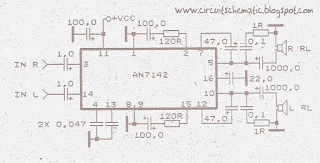This is schematic circuit and i design in simple circuit. Click to view Enlarge
AN7133
This circuit is stereo power amplifier . Voltage input or Supply voltage with dual voltage minimum 6 volt , and maximum 18 volt . You can't  use triple voltage in this amplifier and must DC voltage not AC voltage . Maximum output power 2 X 15 watt  with minimum impedance 4 ohm . You can use this amplifier on car commonly called car amplifier, on Personal Computer (PC) , on small room , etc.
See this schematic amplifier circuit with IC AN7133 below :
AN7120
This schematic use the IC AN7120 for main system power amplifier with support some other components. In this circuit have a good sound quality. Because output noise is very very minimal , not even be heard in the distance of 5 Cm from the sound. Description from this circuit , voltage minimum required 6 volt and maximum voltage 20 volt , you can choose voltage between it. Maximum output Power 15 Watt with impedance 4 Ohm.

See Schematic Power Amplifier below :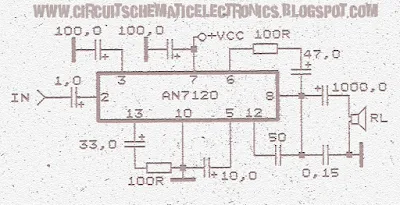(Figure 1.0) Schematic Circuit Power Amplifier AN7120

AN7118S
Although the schematic power amplifier with IC AN7118S is difficult for lees understood making of  PCB track . But once tried to design on PCB is not so difficult. Because the actual components close together and easy to design it. This Circuit need minimum voltage 1 volt and  maximum voltage 3 volt. It is a low voltage amplifier with power output 2 x 35 m Watt .

Schematic circuit below :Click to Enlarge
Components Required :

Resistor / Condensator / Capacitor : 4,7Ohm (2x) , 0,01 uF (2x) , 47uf (2x) , 68 pf (2x) , 1uF (2x) ,  22uF (2x) , 220 uF (1x) , 470uf (2x). Condensator / capacitor voltage use 16 volt. Only Condensator on output speaker use voltage 25 volt.

AN7117
Anyone who uses this amplifier circuit would be surprised. Although this circuit has a small power output just 10 watts , but also issued a sound loud enough. At least match the transistor power amplifier with 60 watts. And his sound is good with low noise. Minimum voltage is 2 volt , and maximum voltage is 12 volt .

Schematic circuit power amplifier AN7117 below :Click image to view Enlarge
AN7116
On this schematic circuit power amplifier with IC AN7116 , this schematic only use the IC because its'not have similiar IC. To operate the Circuit , you must have any component's too , such as resistor , condensator , etc. In order to be a circuit that works on good power amplifier.
This Circuit requires minimum voltage 3 volt and maximum voltage 9 volt . Ouput power of this circuit is 1 watt, and impedance is 4 Ohm.

To see Schematic Circuit ,please see below on  figure 1.0 :Figure 1.0
And see Package IC AN7116 below :This package of IC : SIL-9
AN7114 AN7115
This Circuit must use the IC AN7114 or you can use the IC AN7115 . Minimum voltage for this IC is 6 Volt and maximum voltage is 11 . You can supply this Circuit with 12 Volt if  you use IC AN7115 because its maximum voltage 13 volt. Output Power 1 watt with Impedance 4 ohm .

See the Schematic (figure 2.0) and package IC (figure 1.0) :Figure 1.0Figure 2.0
AN7112
Schematic Circuit use the IC AN7112 with minimum voltage 4 Volt and maximum voltage 14 Volt. Recomeneded voltage is 9 Volt. Maximum output power  0,5 watt. This is low output power or mini amplifier sound. Impedance Speaker 8 Ohm and frequncy 40 Hz - 18 kHz. IC AN7112 has similarities with IC KA2212 , LA4140 , TA7313AP . PAckage of this IC is SIL - 9 and Manufactered by MATSUSHITA.
See Schematic power amplifier (figure 1.1) and Package IC (figure 1.0)  below :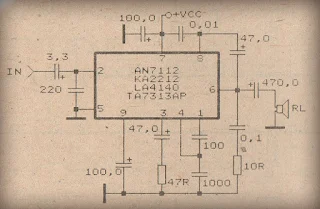Figure 1.1 Click Image to View EnlargeFigure 1.0
AN7111
Schematic Circuit Amplifier with IC AN7111 . This Circuit is a minimum voltage 6 Volt and maximum voltage 18 Volt . And I recomended voltage for this circuit is 12 Volt. With IC AN7111 maximum output speaker 1,2 Watt , and impedance 8 Ohm. Similarity with this IC is AN7131 and AN7140. Package IC : SIL-9 and manufactered by MATSUSHITA.

See  Schematic and package IC below :Click Image to view Enlarge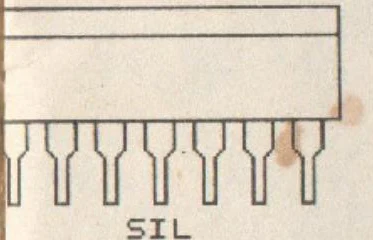This Figure of package IC
AN7110 / AN7130
Description  Audio Power Amplifier with IC AN7110 / AN7130 :
Minimum Voltage for this Circuit is 6 Volt , and Maximum voltage is 18 Volt . To recomended circuit working use voltage 12 Volt. Its not So much and less , its good. Output speaker is Mono with power 1,2 watt and Impedance 8 Ohm. IC equal with AN7130.
See schematic circuit audio power amplifier AN7110 or AN7130 :Circuit diagram  Audio Power Amplifier with IC AN7110 / AN7130
AN7106K
This Schematic must use the IC AN7106K because its'nt similarity, and some components. Minimum voltage is 1,8 Volt and Maximum voltage is 4,8 volt . It,s so very low voltage require. Recomended voltage is 3 volt , don't provide voltage too high it can make damage IC. Maximal Output 2 X 0,14 watt , this is stereo amplifier. And impedance is 32 Ohm.

See Schematic and package IC AN7106K below :Package IC DIP24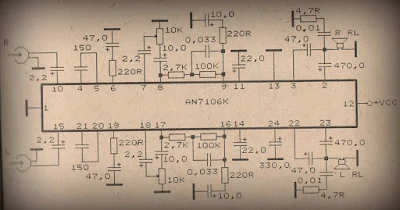Click Image to view enlarge.
AN7105
This Schematic Circuit Audio Amplifier use IC AN7105 , minimum voltage 1,5 volt and maximum voltage 9,5 volt. This is a low voltage Amplifier , with output speaker stereo 2 X 0,38 watt . Its so low power amplifier. Impedance speaker is 32 Ohm ,with Frequency 20 Hz -20 kHz.

See this Circuit and package IC AN7105 below :Package IC is DIP-18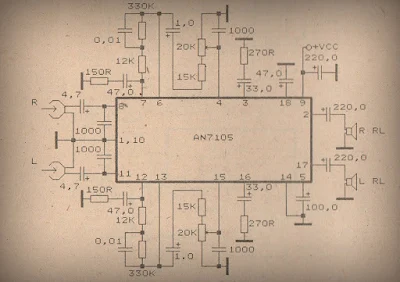Click to view Enlarge

AN7102S
This Schematic use IC AN7102S , this is setereo amplifier and have output speaker 7,5 mW with impedance 32 Ohm . Supply need 1Volt to 3 Volt , wow this amplifier low voltage. You can use this amplifier on radio is use battery . With just one battery with voltage 1,5 Volt you can play this amplifier.
See this Schematic below :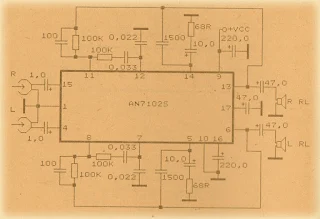Click to Enlarge

Datasheet IC AN7102S
Vcc                   =  1-3 V
Pout                 = 2X 7,5 mW
RL                    = 32 Ohm
Ft                      = 20hz - 20Khz
Icco                  = 7 mA
Package         = DIP-18
Manufactered = MATSUSHITA

AN272
Description for this Schematic :
Power Supply need 16 to 34 Volt , this is very beautiful voltage because with voltage 16 -34 Volt will  be strong to make a loud sound. IC need AN272 ,this ic no similarities. You must use this IC. Ouput speaker Mono , with Power 5W and Impedance 8 Ohm.Click to Enlarge

Datasheet IC AN272
Vcc                = 16-34 V
Pout               =5 W
RL                  = 8 Ohm
Ft                   = 40hz - 18Khz
Icco                = 20 mA
Package         = SIP2-10
Manufactered = MATSUSHITA

AN374
This Schematic use IC AN374, this is mono amplifier and have output speaker 1 W with impedance 8 Ohm . Supply need 8Volt to 16 Volt , this Schematic you can use Voltage 12 V. And better use the stabilizer with 7812 let the voltage stabil. The Effect will be on the sound so smooth.
See this Schematic below  and see datasheet IC AN734 :

Datasheet IC AN374
Vcc                = 8-16 V
Pout               = 1 W
RL                  = 8 Ohm
Ft                   = 50hz - 17Khz
Icco                = 12 mA

AN5260
This is a simple circuit schematic, you can string up this circuit easily. You just need IC AN5260 with some components such as , Condensator Electrolit , Resistor , Ceramic Condensator . Schematic require voltage 12 to 26 Volt . And i use current voltage of 12 volt. Its nice sound , smooth , and low noise amplifier. You want to try this schematic ? .
See this schematic below :

Datasheet IC 5260
Vcc                = 12-26 V
Pout               = 6,6 W
RL                  = 8 Ohm
Ft                   = 30hz - 18Khz
Icco                = 60 mA
Package         = SIP2-11
Manufactered = MATSUSHITA

AN5265
Schematic Description :
This Circuit Require voltage 12 -26 Volt. Good Voltage use is 16 Volt , Because its not too small and not too big Voltage. If use voltage that is to large it will quickly damage the IC. And if use voltage that is to small it will make less than maximum sound output.
See this Circuit  and datsheet IC AN5265 Below :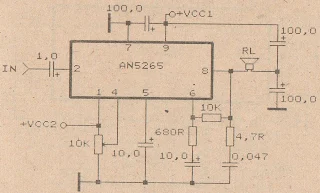Click to Enlarge

Datasheet IC  AN5265
Vcc                = 12-26 V
Pout               = 2,3 W
RL                  = 16 Ohm
Ft                   = 40hz - 18Khz
Icco                = 9,5mA
Package         = SIP2-9
Manufactered =  MATSUSHITA

AN313
This schematic very suitable applied on small speakers. Because the maximum output is 3W and the minimum voltage is 12 Volt  , this will make speaker does not damage quickly. If you use speaker with impedance 8 Ohm , you must make parallel circuit speaker don't use series circuit.
See This Schematic and datasheet IC AN313 below :

Datasheet IC AN313
Vcc                = 12-20 V
Pout               =  2x3 W
RL                  = 8 Ohm
Ft                   = 40hz -18Khz
Icco                = 40 mA
Package         = TABS6-16

AN315
This Schematic Require voltage 9 - 18 Volt. Good voltage for this schematic is 12 V. This is Mono amplifier with output 5,5 Watt and impedance 8 Ohm. You can make this Circuit easily because i give simple and clear scheme.
See this Schematic and Datsheet IC AN315Click to Enlarge

Datasheet IC AN315
Vcc                = 9-18 V
Pout               = 5,5 W
RL                  = 8 Ohm
Ft                   = 30hz - 18Khz
Icco                = 28 mA
Package         = SIP2-11
Manufactered = MATSUSHITA

AN214
See the circuit IC AN214 Audio Power Amplifier below:

Datasheet IC AN214
Vcc                = 6-18 V
Pout               = 4,4W
RL                  = 4 Ohm
Ft                   = 50hz - 17Khz
Icco                = 20 mA
Package         = SIP2-9
Manufactered = MATSHUSHITA

AN7108
AN7108 amplifier See this circuit below:
Datasheet IC AN7108
Vcc                = 1-6,6 V
Pout               = 2X 30 mW
RL                 = 32 Ohm
Ft                   = 20-20 Khz
Icco               = 14 mA
Package         = DIP-16
Manufactered = MATSUSHITA